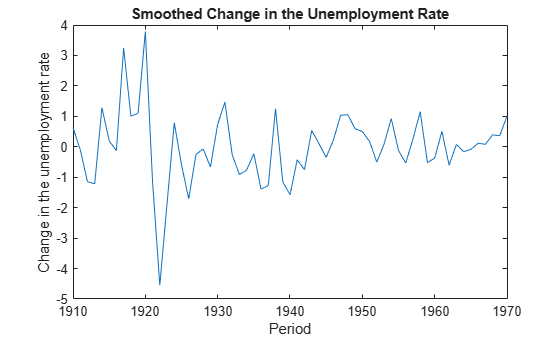Documentation

This is machine translation

Mouseover text to see original. Click the button below to return to the English version of the page.

Smooth States of State-Space Model Containing Regression Component

This example shows how to smooth states of a time-invariant, state-space model that contains a regression component.

Suppose that the linear relationship between the change in the unemployment rate and the nominal gross national product (nGNP) growth rate is of interest. Suppose further that the first difference of the unemployment rate is an ARMA(1,1) series. Symbolically, and in state-space form, the model is

`$\begin{array}{l}\left[\begin{array}{c}{x}_{1,t}\\ {x}_{2,t}\end{array}\right]=\left[\begin{array}{cc}\varphi & \theta \\ 0& 0\end{array}\right]\left[\begin{array}{c}{x}_{1,t-1}\\ {x}_{2,t-1}\end{array}\right]+\left[\begin{array}{c}1\\ 1\end{array}\right]{u}_{1,t}\\ {y}_{t}-\beta {Z}_{t}={x}_{1,t}+\sigma {\epsilon }_{t},\end{array}$`

where:

• ${x}_{1,t}$ is the change in the unemployment rate at time t.

• ${x}_{2,t}$ is a dummy state for the MA(1) effect.

• ${y}_{1,t}$ is the observed change in the unemployment rate being deflated by the growth rate of nGNP (${Z}_{t}$).

• ${u}_{1,t}$ is the Gaussian series of state disturbances having mean 0 and standard deviation 1.

• ${\epsilon }_{t}$ is the Gaussian series of observation innovations having mean 0 and standard deviation $\sigma$.

Load the Nelson-Plosser data set, which contains the unemployment rate and nGNP series, among other things.

`load Data_NelsonPlosser`

Preprocess the data by taking the natural logarithm of the nGNP series, and the first difference of each series. Also, remove the starting `NaN` values from each series.

```isNaN = any(ismissing(DataTable),2); % Flag periods containing NaNs gnpn = DataTable.GNPN(~isNaN); u = DataTable.UR(~isNaN); T = size(gnpn,1); % Sample size Z = [ones(T-1,1) diff(log(gnpn))]; y = diff(u);```

Though this example removes missing values, the software can accommodate series containing missing values in the Kalman filter framework.

Specify the coefficient matrices.

```A = [NaN NaN; 0 0]; B = [1; 1]; C = [1 0]; D = NaN;```

Specify the state-space model using `ssm`.

`Mdl = ssm(A,B,C,D);`

Estimate the model parameters. Specify the regression component and its initial value for optimization using the `'Predictors'` and `'Beta0'` name-value pair arguments, respectively. Restrict the estimate of $\sigma$ to all positive, real numbers.

```params0 = [0.2 0.2 0.1]; [EstMdl,estParams] = estimate(Mdl,y,params0,'Predictors',Z,... 'Beta0',[0.2 0.1],'lb',[-Inf,-Inf,0,-Inf,-Inf]);```
```Method: Maximum likelihood (fmincon) Sample size: 61 Logarithmic likelihood: -99.7245 Akaike info criterion: 209.449 Bayesian info criterion: 220.003 | Coeff Std Err t Stat Prob ---------------------------------------------------------- c(1) | -0.34098 0.29608 -1.15164 0.24948 c(2) | 1.05003 0.41377 2.53771 0.01116 c(3) | 0.48592 0.36790 1.32079 0.18657 y <- z(1) | 1.36121 0.22338 6.09358 0 y <- z(2) | -24.46711 1.60018 -15.29023 0 | | Final State Std Dev t Stat Prob x(1) | 1.01264 0.44690 2.26592 0.02346 x(2) | 0.77718 0.58917 1.31912 0.18713 ```

`EstMdl` is an `ssm` model, and you can access its properties using dot notation.

Smooth the states. `EstMdl` does not store the data or the regression coefficients, so you must pass in them in using the name-value pair arguments `'Predictors'` and `'Beta'`, respectively. Plot the smoothed states. Recall that the first state is the change in the unemployment rate, and the second state helps build the first.

```SmoothedX = smooth(EstMdl,y,'Predictors',Z,'Beta',estParams(end-1:end)); figure plot(dates(end-(T-1)+1:end),SmoothedX(:,1)); xlabel('Period') ylabel('Change in the unemployment rate') title('Smoothed Change in the Unemployment Rate')```